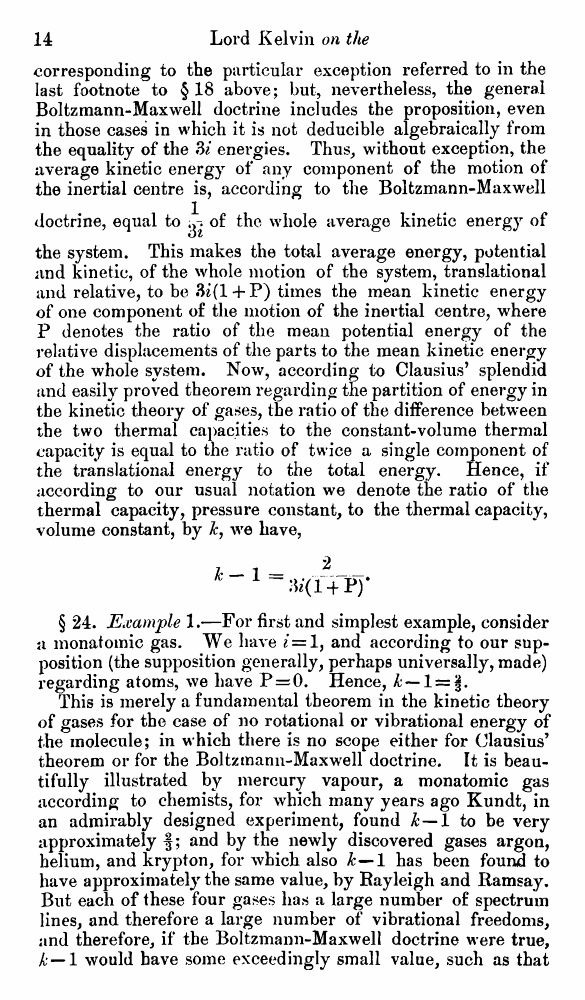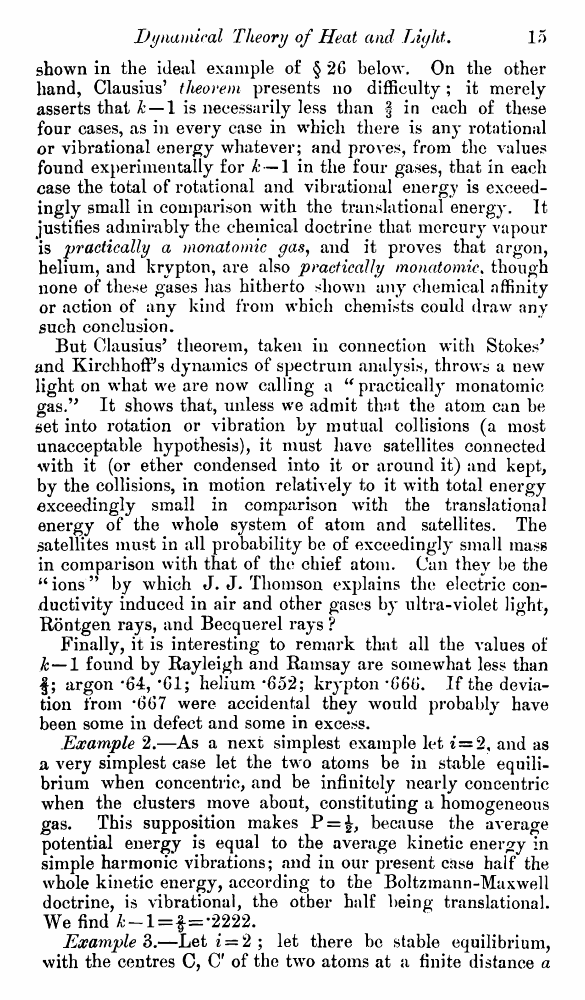# Lord Kelvin. Nineteenth-Century Clouds over the Dynamical Theory of Heat and Light. // Phil. Mag. S. 6. Vol. 2. No. 7. July 1901.

В начало   Другие форматы   <<<     Страница 14   >>>1  2  3  4  5  6  7  8  9  10  11  12  13  14 15  16  17  18  19  20  21  22  23  24  25  26  27  28  29  30  31  32  33  34  35  36  37  38  39  40 corresponding to the particular exception referred to in the last footnote to § 18 above; but, nevertheless, the general Boltzmann-Maxwell doctrine includes the proposition, even in those cases in which it is not deducible algebraically from the equality of the 3i energies. Thus, without exception, the average kinetic energy of any component of the motion of the inertial centre is, according to the Boltzmann-Maxwell doctrine, equal to ^ of the whole average kinetic energy of the system. This makes the total average energy, potential and kinetic, of the whole motion of the system, translational and relative, to be 3i(l + P) times the mean kinetic energy of one component of the motion of the inertial centre, where P denotes the ratio of the mean potential energy of the relative displacements of the parts to the mean kinetic energy of the whole system. Now, according to Clausius’ splendid and easily proved theorem regarding the partition of energy in the kinetic theory of gases, the ratio of the difference between the two thermal capacities to the constant-volume thermal capacity is equal to the ratio of twice a single component of the translational energy to the total energy. Hence, if according to our usual notation we denote the ratio of the thermal capacity, pressure constant, to the thermal capacity, volume constant, by &, we have, t-i- 2-~ 3»(1 + P)‘ § 24. Example 1.—For first and simplest example, consider a monatomic gas. We have i=1, and according to our supposition (the supposition generally, perhaps universally, made) regarding atoms, we have P = 0. Hence, k —1 = §. This is merely a fundamental theorem in the kinetic theory of gases for the case of no rotational or vibrational energy of the molecule; in which there is no scope either for Clausius’ theorem or for the Boltzmann-Maxwell doctrine. It is beautifully illustrated by mercury vapour, a monatomic gas according to chemists, for which many years ago Kundt, in an admirably designed experiment, found A —1 to be very approximately f; and by the newly discovered gases argon, helium, and krypton, for which also Jc—1 has been found to have approximately the same value, by Rayleigh and Ramsay. But each of these four gases has a large number of spectrum lines, and therefore a large number of vibrational freedoms, and therefore, if the Boltzmann-Maxwell doctrine were true, k — 1 would have some exceedingly small value, such as that shown in the ideal example of § 26 below. On the other hand, Clausius’ theorem presents no difficulty; it merely asserts that k — 1 is necessarily less than § in each of these four cases, as in every case in which there is any rotational or vibrational energy whatever; and proves, from the values found experimentally for k — 1 in the four gases, that in each case the total of rotational and vibrational energy is exceedingly small in comparison with the translational energy. It justifies admirably the chemical doctrine that mercury vapour is practically a monatomic gas, and it proves that argon, helium, and krypton, are also practically monatomic. though none of these gases has hitherto shown any chemical affinity or action of any kind from which chemists could draw any such conclusion. But Clausius’ theorem, taken in connection with Stokes' and KirchhofFs dynamics of spectrum analysis, throws a new light on what we are now calling a “ practically monatomic gas.” It shows that, unless we admit that the atom can be set into rotation or vibration by mutual collisions (a most unacceptable hypothesis), it must have satellites connected with it (or ether condensed into it or around it) and kept, by the collisions, in motion relatively to it with total energy exceedingly small in comparison with the translational energy of the whole system of atom and satellites. The satellites must in all probability be of exceedingly small mass in comparison with that of the chief atom. Can they be the “ions” by which J. J. Thomson explains the electric conductivity induced in air and other gases by ultra-violet light, Rontgen rays, and Becquerel rays ? Finally, it is interesting to remark that all the values of k — 1 found by Rayleigh and Ramsay are somewhat less than f; argon ‘64, *61; helium *652; krypton *666. If the deviation from #667 were accidental they would probably have been some in defect and some in excess. Example 2.—As a next simplest example let 2, and as a very simplest case let the two atoms be in stable equilibrium when concentric, and be infinitely nearly concentric when the clusters move about, constituting a homogeneous gas. This supposition makes P = £, because the average potential energy is equal to the average kinetic energy in simple harmonic vibrations; and in our present case half the whole kinetic energy, according to the Boltzmann-Maxwell doctrine, is vibrational, the other half being translational. We find —l = f=-2222. Example 3.—Let i = 2 ; let there be stable equilibrium, with the centres C, C' of the two atoms at a finite distance a